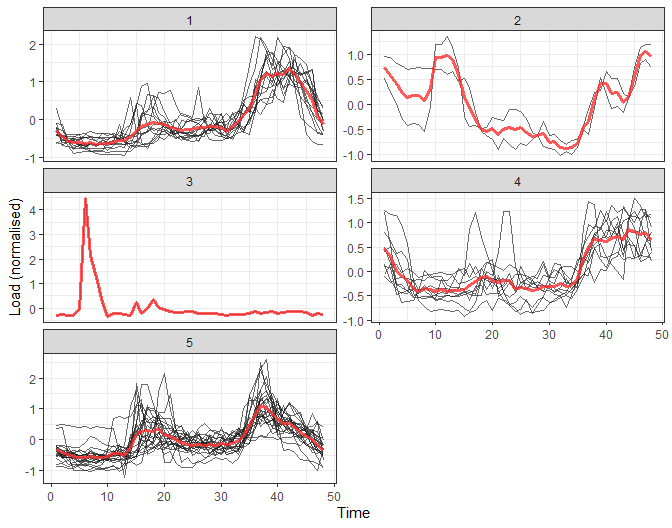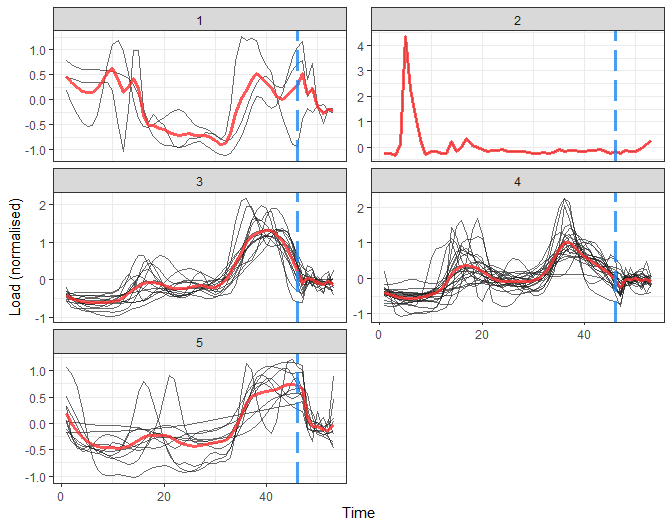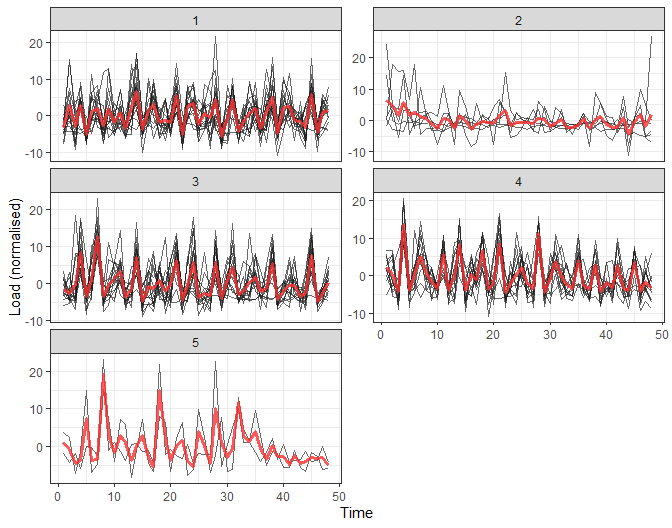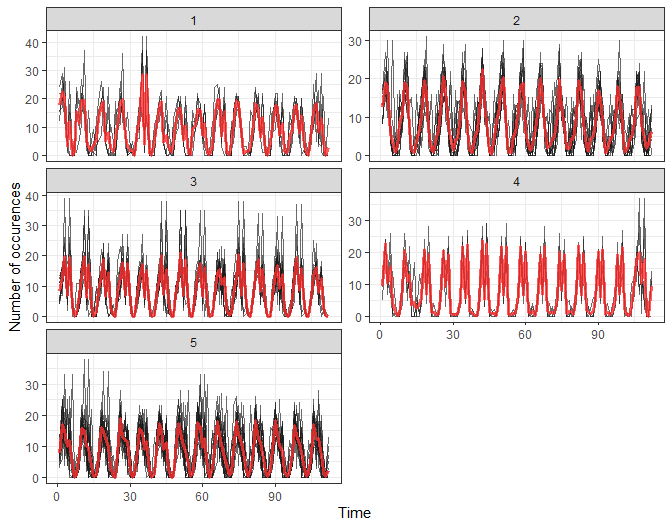TSrepr: Time series representations in a use case

2020-07-12

In this vignette (tutorial), I will show you one use case to use time series representations effectively. This use case is clustering of time series and it will be clustering of consumers of electricity load.

Clustering of time series representations

By clustering of consumers of electricity load, we can extract typical load profiles, improve the accuracy of consequent electricity consumption forecasting, detect anomalies or monitor a whole smart grid (grid of consumers) (Laurinec et al. (2016), Laurinec and Lucká (2016), Laurinec and Lucká (2018)). I will show you the first use case, the extraction of typical electricity load profiles by K-means clustering method.

Firstly, let’s load the required packages, data (elec_load) and return the dimensions of the dataset.

library(TSrepr)
library(ggplot2)
library(data.table)

##   50 672

There are 50 time series of length 672, specifically time series of length 2 weeks. It is obvious that dimensionality is too high and the curse of dimensionality can happen. For this reason, we have to reduce dimensionality in some way. One of the best approaches is to use time series representations in order to reduce dimensionality, reduce noise and emphasize the main characteristics of time series.

For double seasonal time series of electricity consumption (daily and weekly seasonality), the model-based representation approaches seem to have best ability to extract typical profiles. However, I will use some other representations too.

Let’s use one of the basic model-based representation methods - mean seasonal profile. It is implemented in the repr_seas_profile function and we will use it alongside repr_matrix function that computes representations for every row of a matrix of time series. One more very important notice here, normalisation of time series is a necessary procedure before every clustering or classification of time series. It is due to a fact that we want to extract typical curves of consumption and don’t cluster based on an amount of consumption. By using the TSrepr package, we can do it all in one function - repr_matrix. We will use z-score normalisation implemented in norm_z function.

data_seasprof <- repr_matrix(elec_load, func = repr_seas_profile,
args = list(freq = 48, func = mean),
normalise = TRUE, func_norm = norm_z)
dim(data_seasprof)
##  50 48

We can see that dimensionality was in fact reduced significantly. Now, let’s use the K-means (kmeans function) clustering method to extract typical consumption profiles. I will set the number of clusters to 5.

res_km <- kmeans(data_seasprof, 5, nstart = 10)

Let’s plot the results of clustering.

# prepare data for plotting
data_plot <- melt(data.table(ID = 1:nrow(data_seasprof),
class = res_km$cluster, data_seasprof), id.vars = c("ID", "class"), variable.name = "Time", variable.factor = FALSE ) data_plot[, Time := as.integer(gsub("V", "", Time))] # prepare centroids centers <- melt(data.table(ID = 1:nrow(res_km$centers),
class = 1:nrow(res_km$centers), res_km$centers),
id.vars = c("ID", "class"),
variable.name = "Time",
variable.factor = FALSE
)

centers[, Time := as.integer(gsub("V", "", Time))]

# plot the results
ggplot(data_plot, aes(Time, value, group = ID)) +
facet_wrap(~class, ncol = 2, scales = "free_y") +
geom_line(color = "grey10", alpha = 0.65) +
geom_line(data = centers, aes(Time, value), color = "firebrick1", alpha = 0.80, size = 1.2) +
labs(x = "Time", y = "Load (normalised)") +
theme_bw()We can see 4 typical extracted profiles - red lines (centroids of clusters). Now, let’s try some more sophisticated method for the extraction of seasonal profiles - GAM regression coefficients. By repr_gam function, we can extract double seasonal regression coefficients - daily and weekly. However, the number of weekly seasonal regression coefficients will be only 6, because the number of the second seasonality coefficients is set to freq_2 / freq_1, so 48*7 / 48 = 7, and 7 - 1 = 6 because of splines computation (differentiation). I will again use repr_matrix function.

data_gam <- repr_matrix(elec_load, func = repr_gam, args = list(freq = c(48, 48*7)),
normalise = TRUE, func_norm = norm_z)
dim(data_gam)
##  50 53

So the dimension is 47 + 6 = 53 because of usage of splines in GAM method. Let’s cluster the data and visualize it.

res_km <- kmeans(data_gam, 5, nstart = 10)
# prepare data for plotting
data_plot <- melt(data.table(ID = 1:nrow(data_gam),
class = res_km$cluster, data_gam), id.vars = c("ID", "class"), variable.name = "Time", variable.factor = FALSE ) data_plot[, Time := as.integer(gsub("V", "", Time))] # prepare centroids centers <- melt(data.table(ID = 1:nrow(res_km$centers),
class = 1:nrow(res_km$centers), res_km$centers),
id.vars = c("ID", "class"),
variable.name = "Time",
variable.factor = FALSE
)

centers[, Time := as.integer(gsub("V", "", Time))]

# plot the results
ggplot(data_plot, aes(Time, value, group = ID)) +
facet_wrap(~class, ncol = 2, scales = "free_y") +
geom_line(color = "grey10", alpha = 0.65) +
geom_line(data = centers, aes(Time, value), color = "firebrick1", alpha = 0.80, size = 1.2) +
geom_vline(xintercept = 46, color = "dodgerblue2", size = 1.4, linetype = 5, alpha = 0.8) +
labs(x = "Time", y = "Load (normalised)") +
theme_bw()That is a more delightful result, isn’t it? Extracted consumption profiles are smoother than in the case of average seasonal profiles. The blue dashed line borders daily and weekly seasonal coefficients.

I will show you also result of clustering of some nondata adaptive representation, let’s pick for example DFT method (Cooley and Tukey (1965)) and extract first 48 DFT coefficients.

data_dft <- repr_matrix(elec_load, func = repr_dft, args = list(coef = 48),
normalise = TRUE, func_norm = norm_z)
dim(data_dft)
##  50 48
res_km <- kmeans(data_dft, 5, nstart = 10)
# prepare data for plotting
data_plot <- melt(data.table(ID = 1:nrow(data_dft),
class = res_km$cluster, data_dft), id.vars = c("ID", "class"), variable.name = "Time", variable.factor = FALSE ) data_plot[, Time := as.integer(gsub("V", "", Time))] # prepare centroids centers <- melt(data.table(ID = 1:nrow(res_km$centers),
class = 1:nrow(res_km$centers), res_km$centers),
id.vars = c("ID", "class"),
variable.name = "Time",
variable.factor = FALSE
)

centers[, Time := as.integer(gsub("V", "", Time))]

# plot the results
ggplot(data_plot, aes(Time, value, group = ID)) +
facet_wrap(~class, ncol = 2, scales = "free_y") +
geom_line(color = "grey10", alpha = 0.65) +
geom_line(data = centers, aes(Time, value), color = "firebrick1", alpha = 0.80, size = 1.2) +
labs(x = "Time", y = "Load (normalised)") +
theme_bw()The interpretability of these results would be difficult. Therefore, model-based time series representations are very effective in this use case.

I will show you the usage of one more representation method - FeaClip. The FeaClip is feature extraction method from a clipping representation (Laurinec and Lucká (2018)). The windowing approach alongside FeaClip is recommended to use for every day of time series. The big advantage is that normalisation is not needed alongside of FeaClip method. Let’s use it in our case.

data_feaclip <- repr_matrix(elec_load, func = repr_feaclip, windowing = TRUE, win_size = 48)
dim(data_feaclip)
##   50 112
res_km <- kmeans(data_feaclip, 5, nstart = 10)
# prepare data for plotting
data_plot <- melt(data.table(ID = 1:nrow(data_feaclip),
class = res_km$cluster, data_feaclip), id.vars = c("ID", "class"), variable.name = "Time", variable.factor = FALSE ) data_plot[, Time := as.integer(gsub("V", "", Time))] # prepare centroids centers <- melt(data.table(ID = 1:nrow(res_km$centers),
class = 1:nrow(res_km$centers), res_km$centers),
id.vars = c("ID", "class"),
variable.name = "Time",
variable.factor = FALSE
)

centers[, Time := as.integer(gsub("V", "", Time))]

# plot the results
ggplot(data_plot, aes(Time, value, group = ID)) +
facet_wrap(~class, ncol = 2, scales = "free_y") +
geom_line(color = "grey10", alpha = 0.65) +
geom_line(data = centers, aes(Time, value),
color = "firebrick1", alpha = 0.80, size = 1.2) +
labs(x = "Time", y = "Number of occurences") +
theme_bw()It seems like good separability of clusters, better than in the case of DFT.

In this tutorial, I showed you the usage of time series representation methods to create more accurate characteristic profiles of consumers.

Bibliography

Cooley, James W, and John W Tukey. 1965. “An Algorithm for the Machine Calculation of Complex Fourier Series.” Mathematics of Computation 19 (90): 297–301.

Laurinec, Peter, Marek Lóderer, Petra Vrablecová, Mária Lucká, Viera Rozinajová, and Anna Bou Ezzeddine. 2016. “Adaptive Time Series Forecasting of Energy Consumption Using Optimized Cluster Analysis.” In Data Mining Workshops (Icdmw), 2016 Ieee 16th International Conference on, 398–405. IEEE.

Laurinec, Peter, and Mária Lucká. 2016. “Comparison of Representations of Time Series for Clustering Smart Meter Data.” In Lecture Notes in Engineering and Computer Science: Proceedings of the World Congress on Engineering and Computer Science 2016, 458–63.

———. 2018. “Interpretable Multiple Data Streams Clustering with Clipped Streams Representation for the Improvement of Electricity Consumption Forecasting.” Data Mining and Knowledge Discovery, November. https://doi.org/10.1007/s10618-018-0598-2.